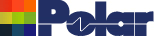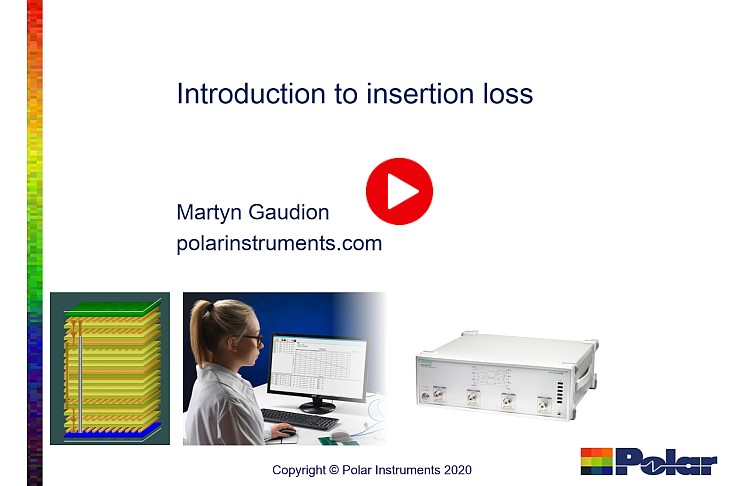November 2020 Webinar video note Introduction to Insertion Loss November 2020 Webinar – Introduction to Insertion LossIntroduction to PCB Insertion Loss Webinar Introduction to PCB insertion loss webinar covering modeling, measuring and a simple to understand explanation of the PCB characteristics that impact on both characteristic impedance and insertion loss of PCB transmission lines. Recorded November 2020. Contents 00:00 Introduction 00:59 The two main contributors to loss 01:45 Copper losses 04:15 Copper roughness 05:25 Dielectric loss 06:50 Modeling loss 07:00 Drivers for Impedance vs Loss 07:40 Factors that influence characteristic impedance 08:50 Factors that influence insertion loss 11:40 "All models are wrong but some are useful" 16:40 Substrate modeling 18:00 Roughness modeling 19:10 Measurement 20:00 Delta-L 4.0 26:25 Atlas insertion loss measurement system 30:31 Contact information